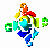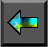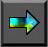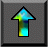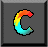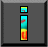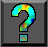Next: Exercises Up: 11 Hypercube Algorithms Previous: 11.4 Mergesort

# 11.5 Summary

The hypercube communication template (Algorithm 11.1) allows information to be propagated among P tasks in just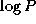steps. Each algorithm considered in this case study has exploited this property to perform some form of all-to-all communication. For example, in matrix transposition each task requires values from every other task; in sorting, the position of each value in the final sequence depends on all other values. Many other parallel algorithms can be naturally formulated in terms of the same template, once the need for all-to-all communication is recognized.

The hypercube template described in this chapter is one of the most useful communication structures in parallel computing. Another useful structure that we have encountered is nearest-neighbor exchange on a two-dimensional torus: this template can be used to implement finite difference computations, matrix multiplication (Section 4.6), and graph algorithms. The manager/worker load balancing structure (Section 2.5.2) is a third example of a template.

Learning to recognize and apply templates such as the hypercube, torus, and manager/worker can greatly simplify the task of designing and implementing parallel programs. When designing a parallel algorithm, we can first seek to formulate communication requirements in terms of known communication structures; if we are successful, the design problem then reduces to that of specifying the application-specific part of the algorithm. A similar strategy can be applied when implementing designs that have been formulated in terms of templates.Next: Exercises Up: 11 Hypercube Algorithms Previous: 11.4 Mergesort